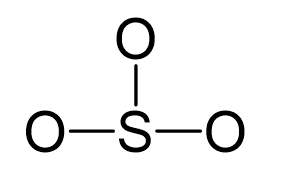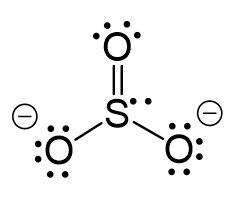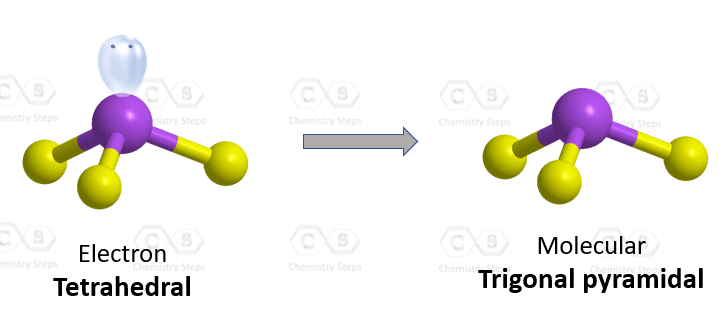## Examples

Sulfur is the central atom:There are 3×6 + 6 + 2 = 26 electrons, and 6 of them are used to make 3 bonds. Three oxygens take 3 lone pairs each and one goes to the sulfur:All the electrons have been used; however, one oxygen needs to share a pair of electrons with the sulfur otherwise the ionic would be -3:The central atom has 3 atoms and a lone pair and therefore, the electron geometry is tetrahedral while the molecular geometry is trigonal pyramidal.Steric number 4 corresponds to sp3-hybridization where the idealized bond angles are 109.5o. Because the groups on the central atom are not identical, the bond angles differ from the idealized values.

More practice examples on geometry and hybridization in this multiple-choice quiz: